## LIDAR BASICS

• LIDAR is based on well known principles of RADAR
• Radar is well known technology existing since the beginning of the 20th century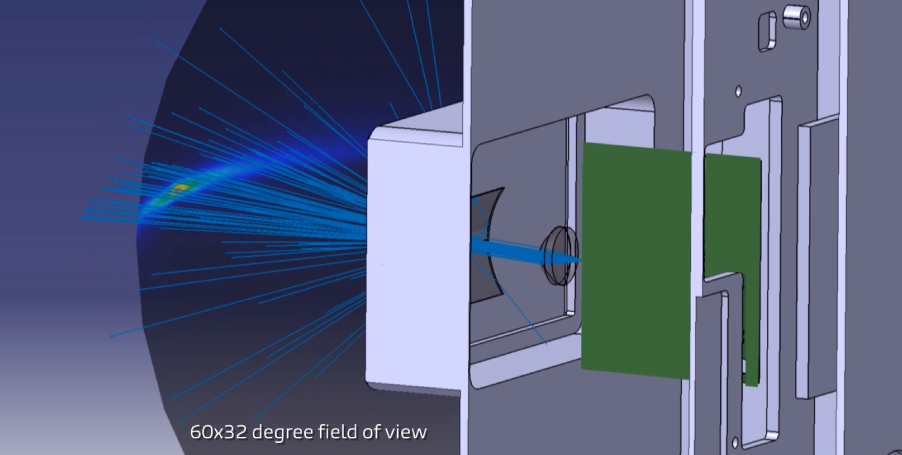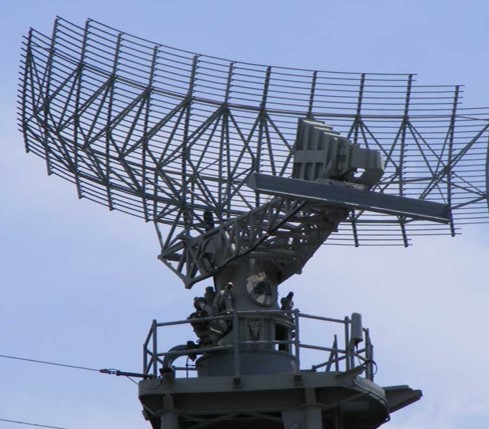### LIDAR using phased arrays improves directionality using interference

• Output profile of a LIDAR is the product of the array factor and the single antenna far field.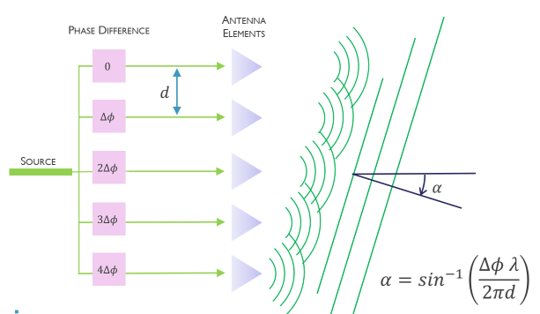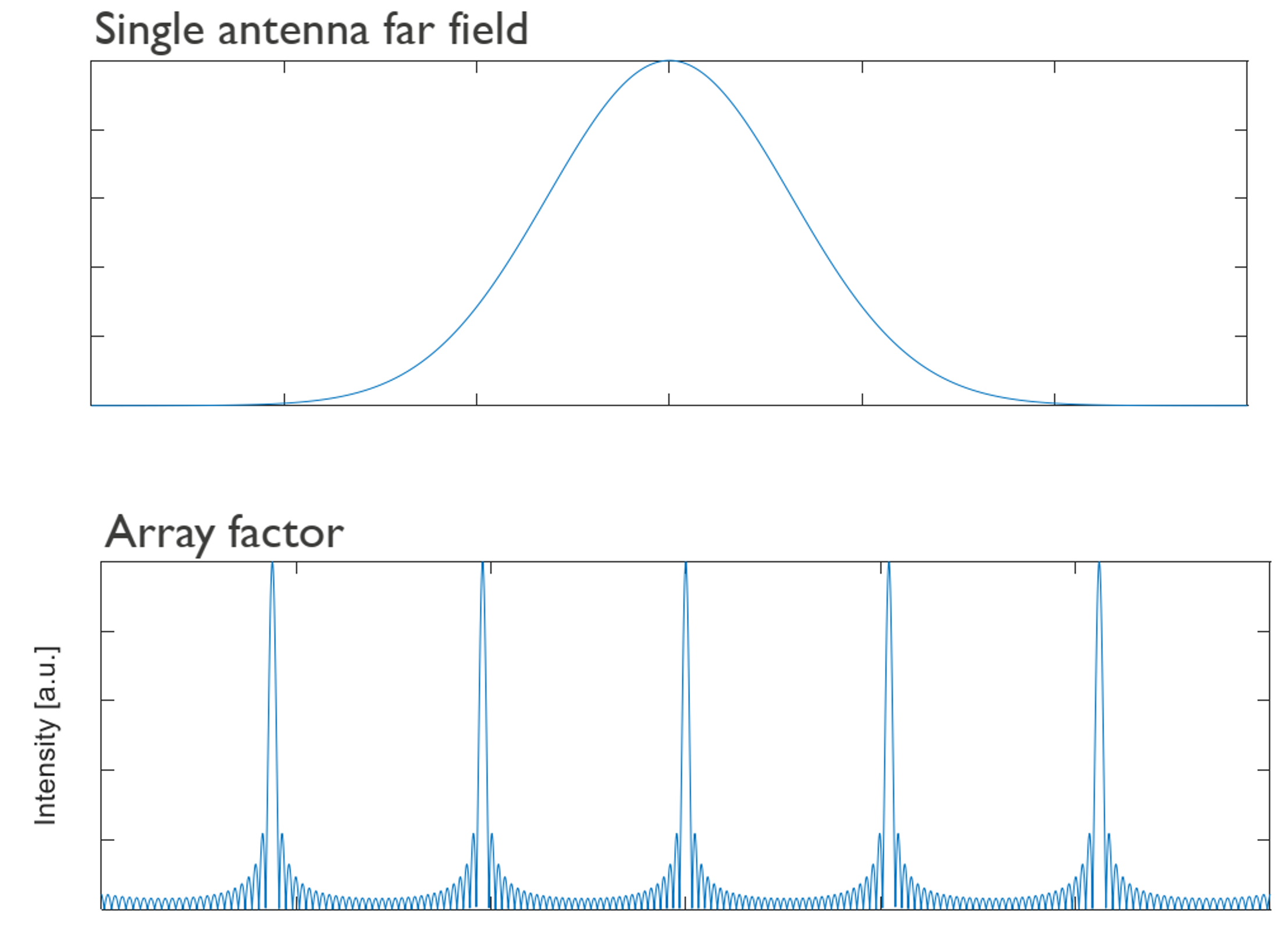N: number of antennas

k: wave vector

w_i: complex weight for element I

r_i: position vector for antenna element i## LIDAR using on-chip waveguide antennas

• Corrugated waveguides are used as antennas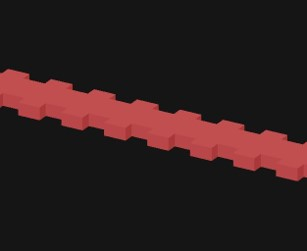• Pitch corrugation is chosen so that Bragg reflected light is scattered to free space• Multiple such antennas are used in an array to for beam forming## Lidar modeling in Lumerical

• MODE analysis of waveguide to calculate n_eff
• Choosing optimum antenna separation so that there is minimum coupling between adjacent antennas
• 3d FDTD simulation to calculate bandgap region
• Far field analysis to calculate far-field profile of a single antenna
• Array factor multiplication to see beam steering

### Mode

Calculate effective refractive index for thinner and thicker cross-sections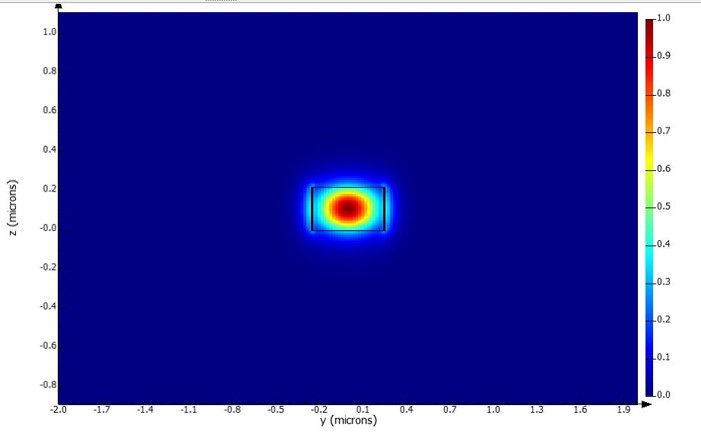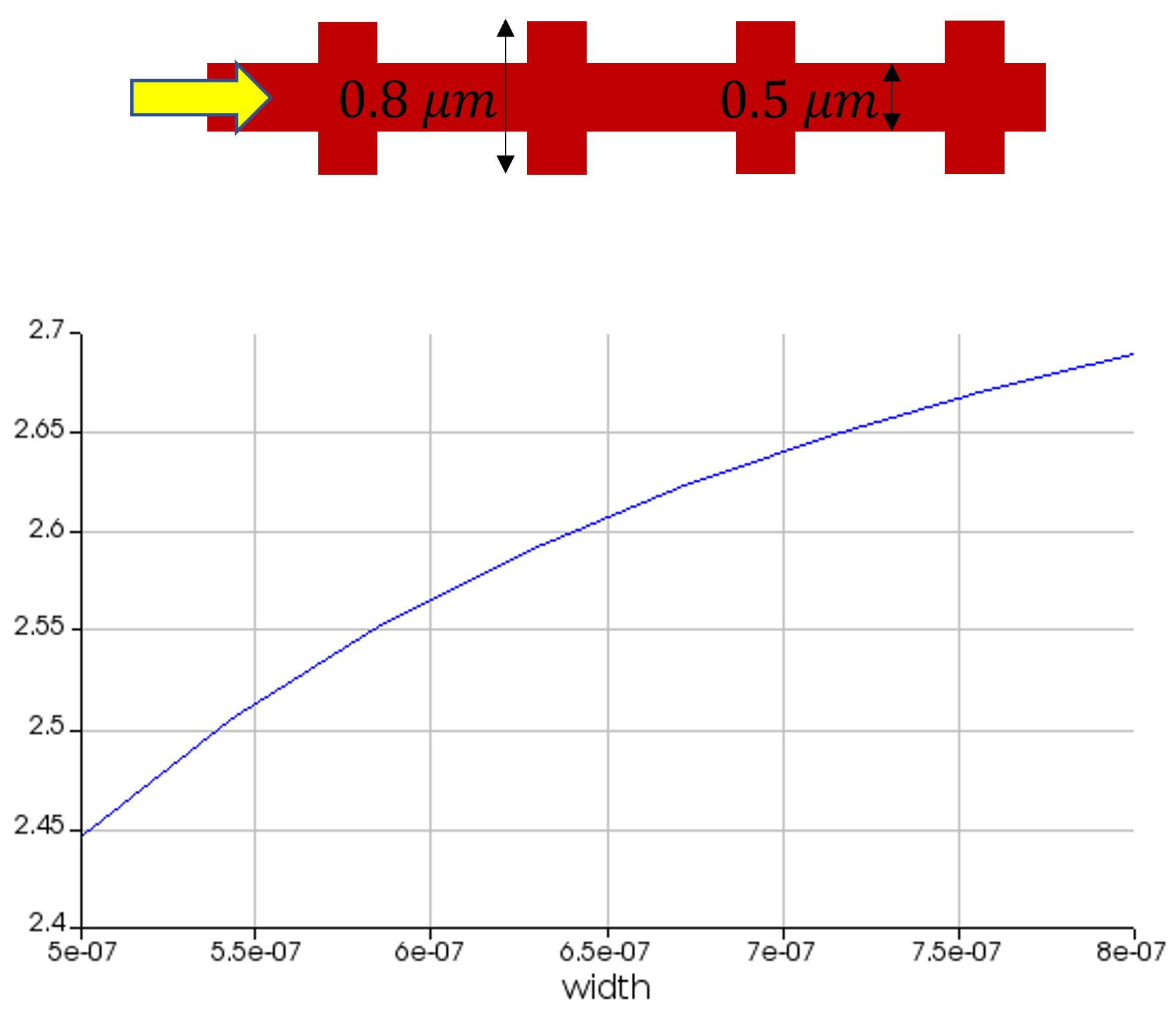• Optimizing separation to minimize cross-coupling
• We perform simulations with 2 waveguides and calculate after how much length can 10% of light from waveguide 1 couple to waveguide 2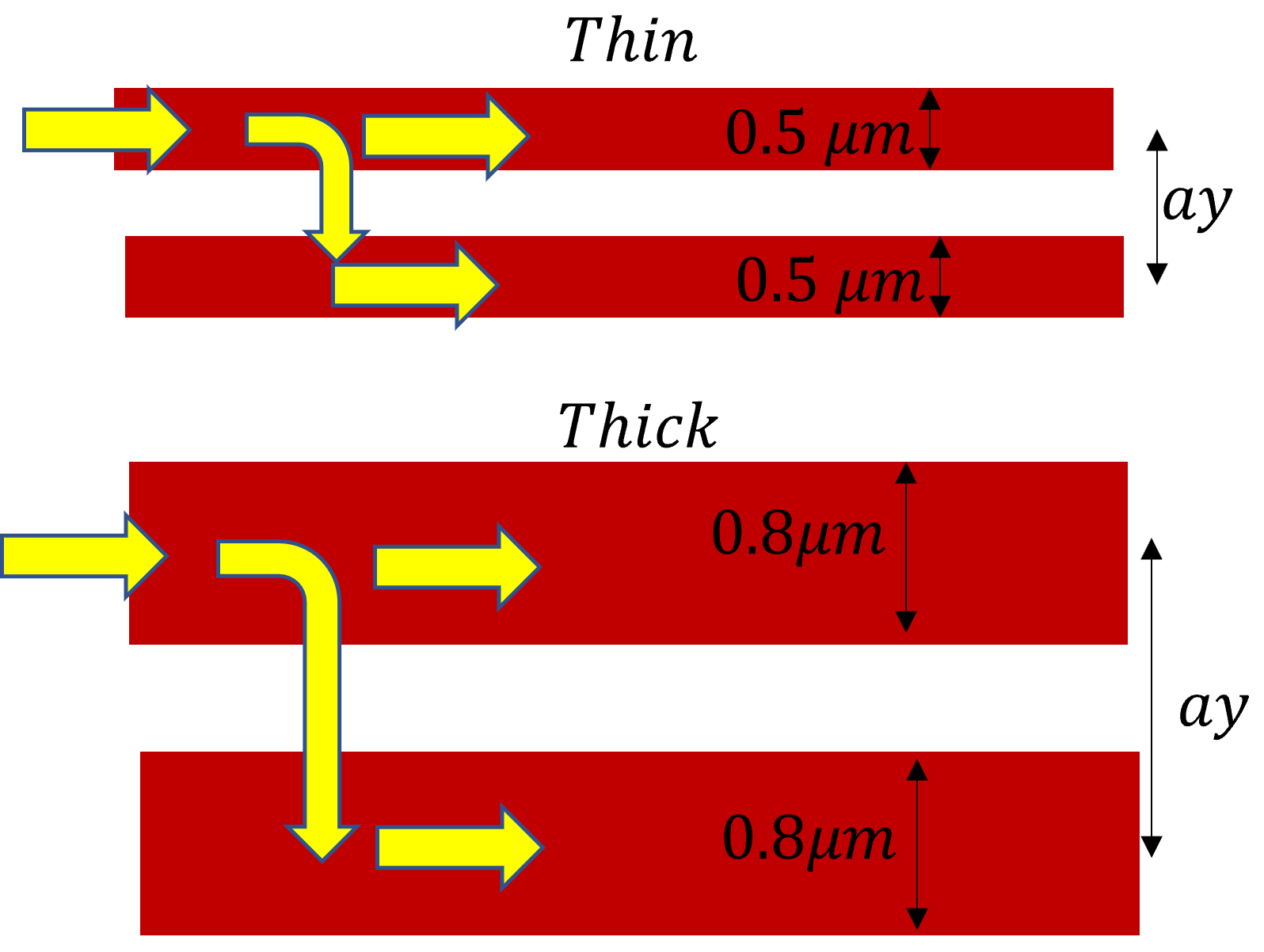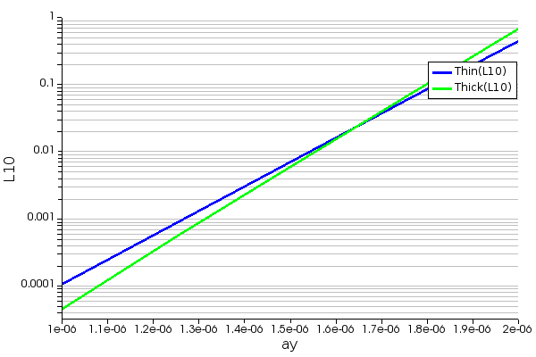### FDTD

• Calculate the band-gap region
• Observe wavelength dependent single antenna scattering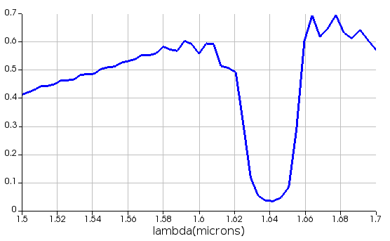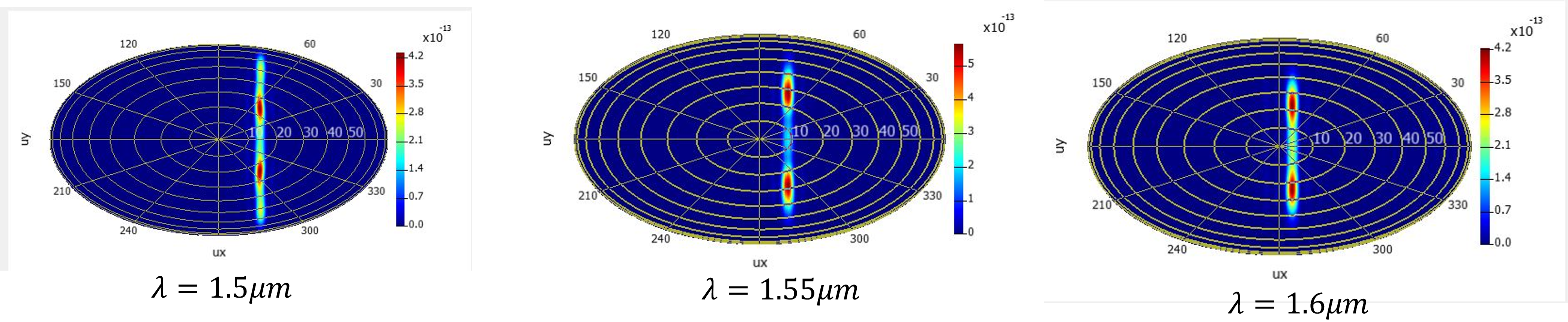• 2d beam-steering(θ,ϕ) using results from FDTD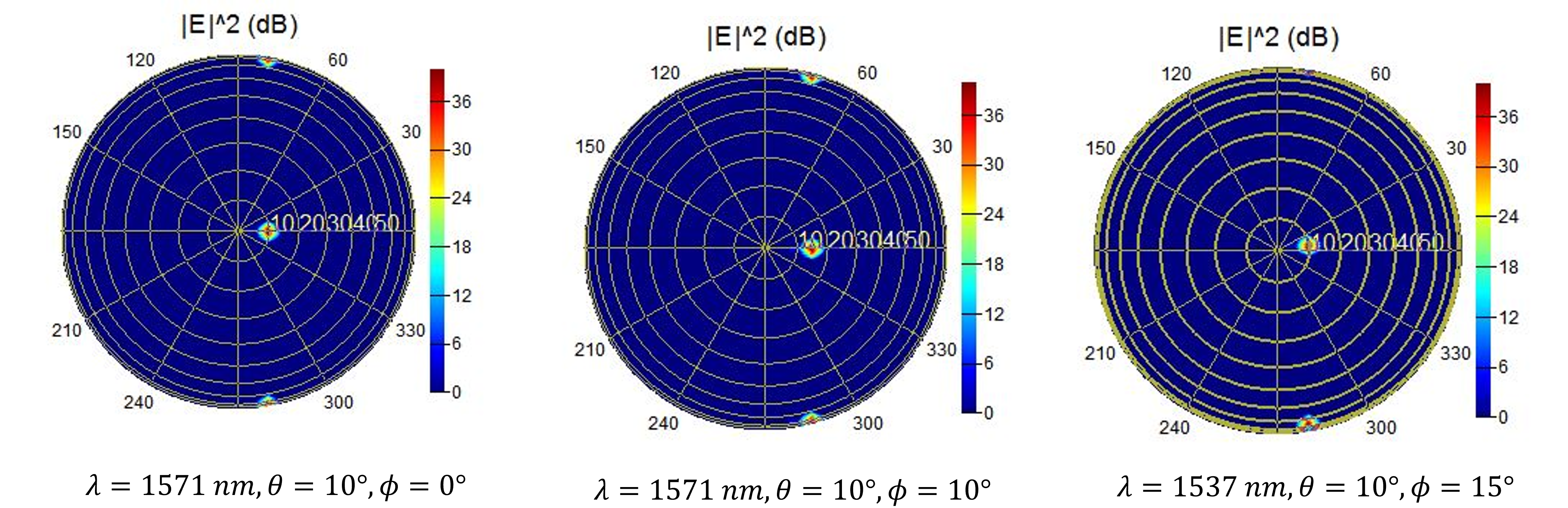## System level simulations using INTERCONNECT

• Integrate multiple components
• Simulate an actual experiment
• Extensive library of standard components
• Custom components can be created
• Allows integration of optical, electrical and thermal elements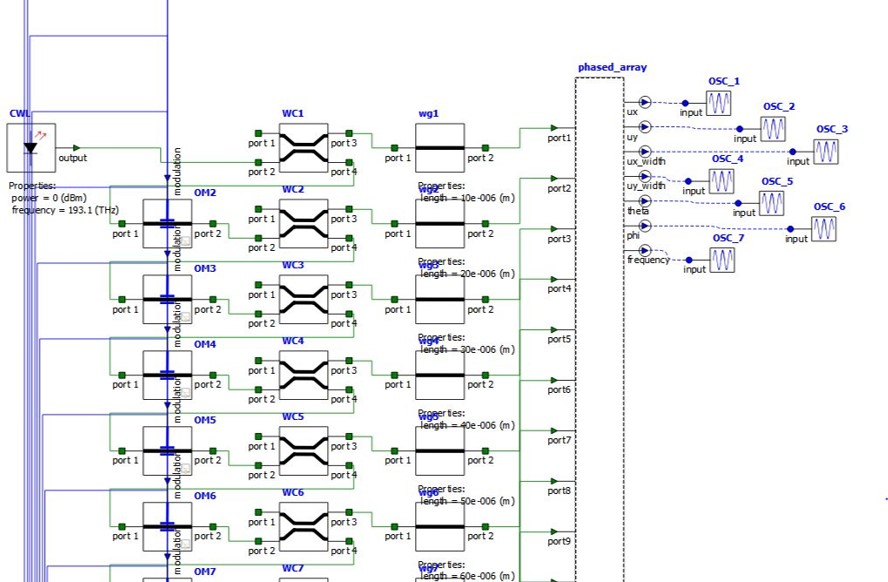• We see the effect of applying a optical delay using a voltage ramp on an optical modulator
• For a given range of voltage values we can monitor θ,ϕ of the steered beam and beam properties.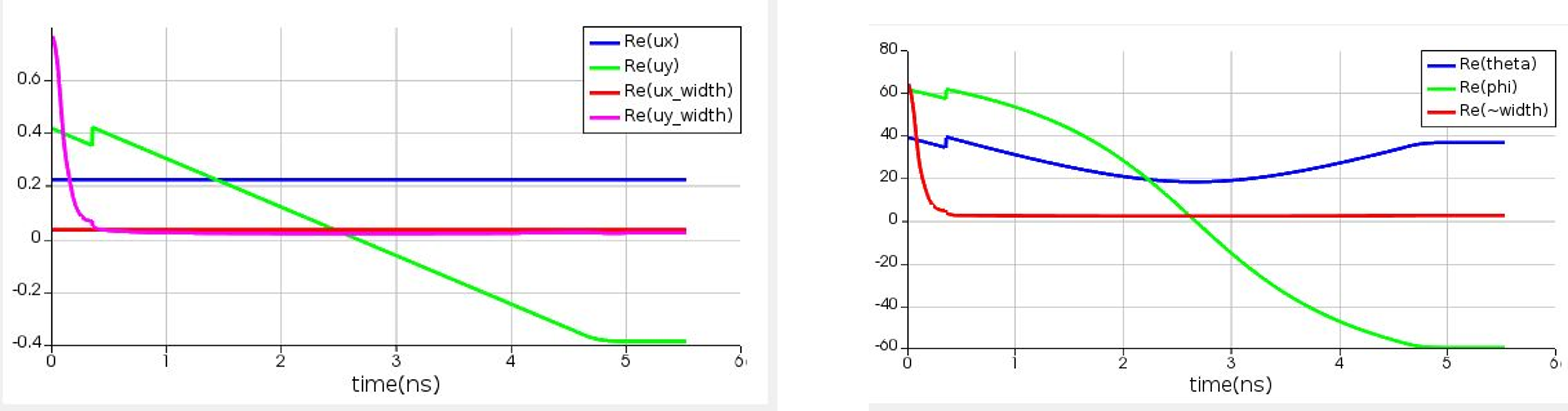## Detector design

• Detector design using Lumerical
• Optical simulations to study light absorption in detectors

Example: We look at Germanium detectors on Si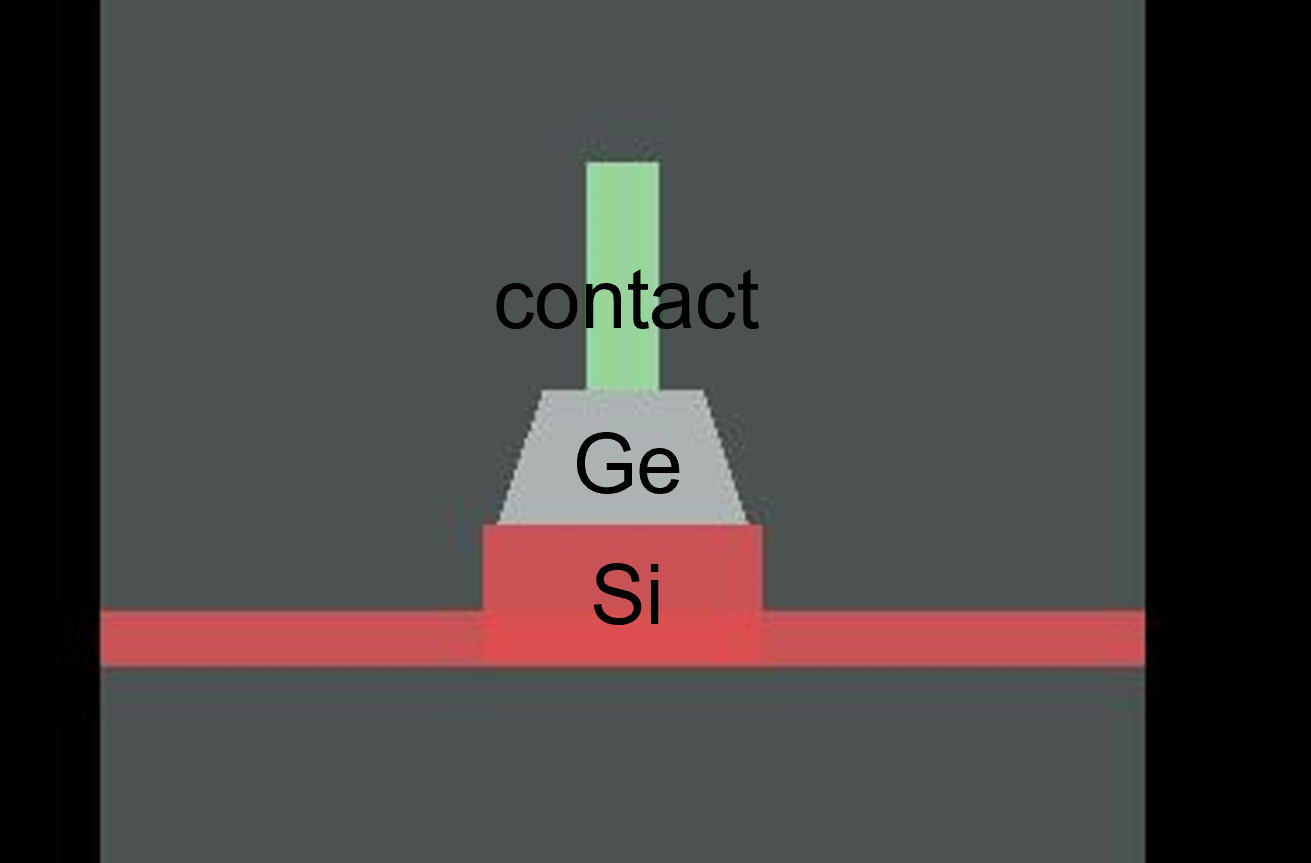Ge on Si detector cross-section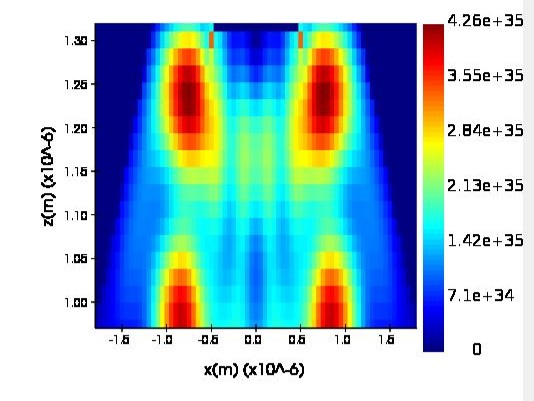Intensity profile in Ge using FDTD

• Electrical simulations using CHARGE can be used to calculate the photocurrent and the dark current for the detector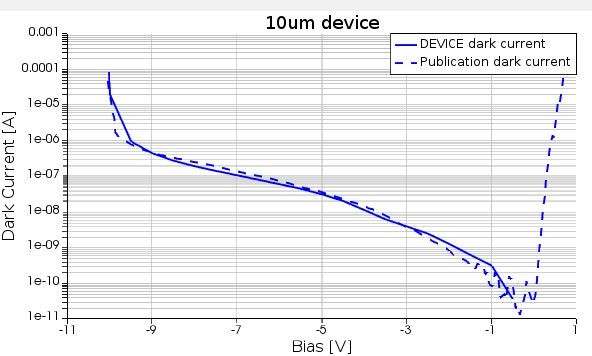• Electrical simulations using CHARGE can be used to calculate the responsivity and gain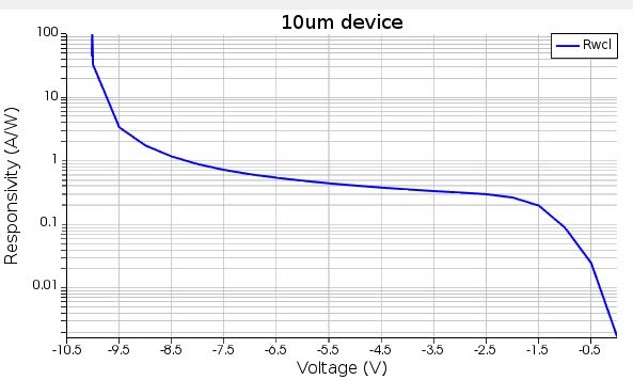• Circuit simulations using INTERCONNECT can be used to simulate detector response to weak modulated signal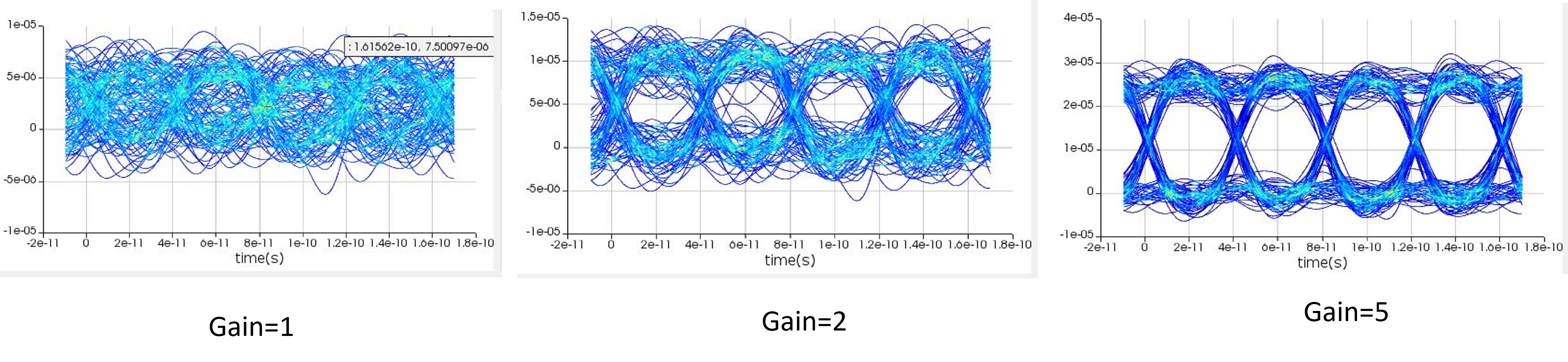###### Tags:
lumerical, photonics, lidarMay 16, 2022